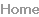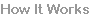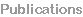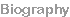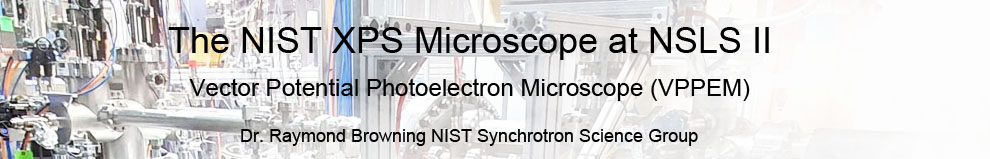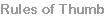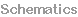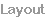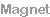Electron  Optics
These pages cover different aspects designing a VPPEM instrumentPage 1
Page 2
 Instrument Design
The electron optics has four relatively independent parts:

1. A magnet, having a ferromagnetic shield with an image
forming aperture.

2. An electrostatic input, or transfer lens.

3. A concentric hemispherical analyser (CHA).

4. An electrostatic output, or imaging forming lens.

These four parts can be usefully modelled independently.
However, as we will show, the image properties are complex,
and using Gaussian optics is not appropriate.

To finalize a design, we need to simulate the magnet, the input
lens, the CHA and the output lens together, and electron
trajectories must be run through the complete system.
Also see:
Rules of Thumb.

All of the field calculations and electron optical trajectory
simulations used Field Precision's 2D TriComp suite.We have built two instruments, one with a liquid helium
temperature magnet having a large 1.25x1.25x1.5 meter
independent ferromagnet shield, and another instrument with a
compact 10x10x10 cm HTS solenoid with a closely coupled
electromagnetic yoke.
Magnet

The first instrument was the proof-of-principle, and even though
it produced excellent results, we do not see this as the best
model to follow. Instead, we will use a compact magnet using
high temperature superconducting (HTS) tape.
The calculation of the trajectories through the optical path is
broken into sections passing the output trajectories from one
section as the input trajectories to the next.

The magnetic and electric fields of the magnetic enclosure and
the input lens are modeled as one unit because it was
necessary to understand the effects of stray fields from the
magnet on the input lens.
 The electron optical design is the core technology in VPPEM.Here we discuss some of the design questions, and show results from the electron optical simulation for the new VPPEM at the NIST beamlines at NSLS II.
To limit to the range of the design parameters used for the input
lens a three element einzel lens with magnification of near unity
was fixed on.

The lens itself  is a 4 element cylindrical electrostatic lens of 60
mm diameter and 450 mm length.

This figure shows the complete magnetic enclosure and the CHA
input lens as a sectional plot with magnetic and electrostatic fields
shown as field lines. The section is rotationally symmetric around
the horizontal axis.
The trajectories in the magnetic enclosure take significant
computer time to process because the synchrotron orbits at low
energies in a strong magnetic field need high precision.

A large set of these trajectories are calculated, and used as inputs
into the input lens which can be designed with less computational
effort.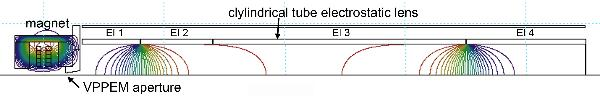Magnetic and electrostatic fields for an HTS compact electromagnet
and a CHA input lens operated as a 3 element einzel lens
A simulation of the HTS magnetic enclosure is shown in here.

The magnetic circuit has an electromagnetic yoke makig a
significant contribution to the central field strength of 2.0 T.

The mild steel yoke is 76 mm diameter, and 48 mm length. The 4
mm aperture insert is pure iron.

The upper right inset  shows a plot of the decay of the central field.
A distinct knee in the decay is created by the presence of the
aperture.

The 50 eV electron trajectories leave the field lines at
approximately 550 Gauss which is slightly into the aperture.

An expanded view of the trajectories is shown the inset upper left.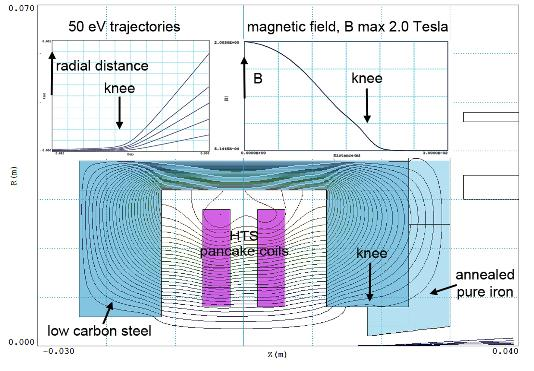In this simulation the fan of trajectories leaving the magnetic field
have started at different off-axis positions at the sample position.

The trajectory plot in the top left insert is deceptively simple, only
the radial distance of the trajectories from the axis is plotted. In
fact, the image is rotated around the axis, and it is not a simple
angular image.In the figure on the right we are looking at a single trajectory down
the magnetic axis.

We see the cyclotron orbit move away from the y axis, and expand
as the field decreases. As the trajectory exits the field, it
undergoes a deflection into its final direction.

Clearly, the final direction will depend on exactly where the orbit
crosses the aperture as the vector potential varies across the
aperture.

Two things to note about the final direction of the trajectory. Firstly,
it is aligned at an angle to the x,y axis, and secondly it does not
cross the x, and y axis in the same place. This is what we expect
as the vector potential is pointing around the axis, and the starting
position of the cyclotron orbit is off axis. The orbit moves further
off-axis as magnetic field weakens.Rather than the simple fan of trajectories shown above where
only the radial distance of the trajectories from the axis is
plotted. If we plot the trajectories in the x, and y directions
separately, We see the image is rotated, and that the image
has its apparent origin on the optical axis in different positions
on the x (the meridional) and the y (the transverse) directions.
The different apparent object positions is a complication when
it comes to focusing the image out of the VPPEM aperture into
the CHA.This is why we view the input lens as a transfer lens,
or as a condenser, because a true focus is not obtainable.

Although we are ultimately using the angular information, the
image coming out of the field aperture is actually a mixture of
spatial, and angular information. The result is that the CHA
input lens does not really ‘focus’ into the CHA. The input lens
produces a non-Gaussian somewhat caustic looking focus.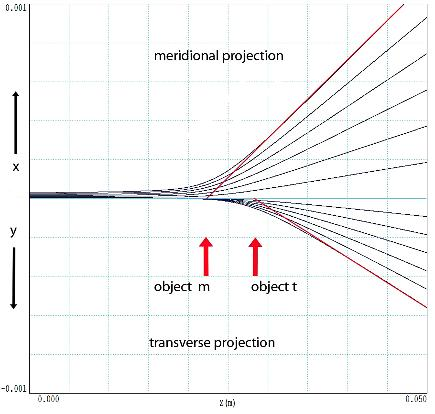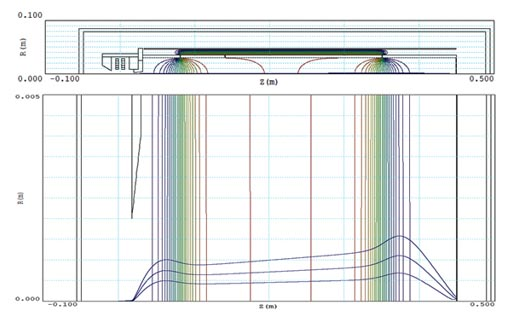This is the CHA input lens shown above expanded.

This figure shows the input lens with three illustrative 50 eV
trajectories. The lens is operated as an accelerating three element
einzel lens for 50 eV electrons with +490 V on the central element.
There is also some focusing from the magnet’s stray field penetrating
into the lens. The ‘focus’ at the exit of the lens is actually a best fit to
minimize the size. This best fit depends on the most extreme
trajectory that will exit through the exit slit of the CHA.

T
he trajectories do not produce a good focus at the input of the CHA,
they are in effect highly aberrated.

However, these apparent aberrations are in
space not in angle.
Similarly, for the intrinsic chromatic aberration of
the CHA. The CHA is
double focusing in θ and φ, however, the energy-dispersed image at
the exit of the CHA is not like the original pinhole image but is spread
out across the CHA output aperture in the dispersion direction.

We can still form a good image with the CHA output lens because we
are focusing on an angular image which is a virtual image at infinity.
The output lens is a telescope with the detector at the prime focus.

Clearly, the fact that the signal is encoded as an angle after leaving
the magnetic field has a large impact on the design of the
electrostatic section of the
VPPEM electron optics.
Trajectories though the CHA were calculated using the CHA equation
of motion. Where R is the center radius of the CHA, α is the half angle
of the electrons entering the CHA, and xi and xo are the distances of
the incoming, and outgoing electron from the central radius R of the
CHA.

Using an Excel spread sheet, and the CHA equation of motion the
final parameters of the input lens trajectories can be converted into
the input trajectory parameters for the output lens. Only those
trajectories that fall within the 0.35 mm output slit of the CHA are
used.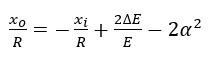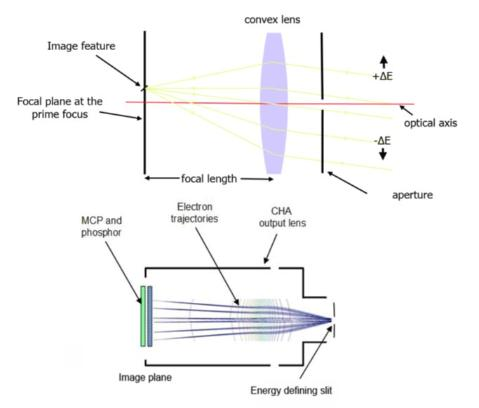An angular electron optical image is very unusual.

The angular image at the exit of the CHA is somewhat like a star field
image. A star field image is made up of parallel beams of light from
sources far away coming in at different angles. A difference here is
that there is energy dispersion across the parallel beams coming out
of the CHA.

The VPPEM output lens is equivalent to a telescope with a small
aperture in front of it. (not exactly the Mount Palomar best practice).
But the aperture chooses one small range of energy.

The angular image is focused as a real image at the prime focus of
the telescope. (where a photo-plate would be in the good old days).
The lens is therefore not focused on the aperture, but at the prime
focus, the image plane.

The aperture can be anywhere, just away from the focusing field, but
not too far, because the lens filling factor increases. The
magnification depends on the focal length. The aberrations depend
on the filling factor. Therefore, it is best to have a long lens with a
large diameter.
Page 3In our case, the output lens is constrained by two geometrical factors.
The first is that the CHA output port has an 8” CF flange, and the
second is the length of the input lens which effectively limits the length
of the output lens before it meets the magnet mounting. The lens
focusses at 380 mm from the CHA output slit.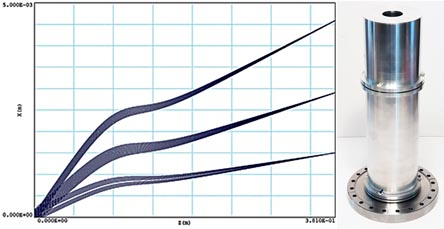Page 4